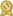Found 3800 calculus tutors – page 8 of 380.

Greenville 27834 · \$40/hour · teaches ACT Math - ADHD - Algebra 1 - Algebra 2 - Chess -

I then went on to teach geometry for one year, and after that, also taught pre-calculus. At this point, after I had completed Calculus I, Calculus II, Calculus III, and Differential equations...certified

Santa Cruz 95060 · \$60/hour · teaches Algebra 2 - Calculus - Discrete Math - Elementary

I have helped students who were struggling with basic algebra to go on and succeed in higher level math classes like Calculus. I tutor Algebra, Pre-Calculus, Trigonometry, Calculus...certified

Dallas 75248 · \$50/hour · teaches Algebra 2 - Calculus - Geometry - Physics -

My expertise is in Geometry,Pre-Algebra, College Algebra, Trigonometry, Pre-Calculus, Calculus, Business Math, Business Calculus, Linear Algebra, Differential Equations,and Physics.certified

Hackensack 07601 · \$60/hour · teaches Ballroom Dancing - Calculus - Elementary Math -

I currently tutor Algebra I, Algebra II, Pre-Calculus, Calculus I, Calculus II courses to Middle/High School and College students. As well, I tutor programming languages such as Assecertified

Irmo 29063 · \$50/hour · teaches Biology - Calculus - Elementary Math - Elementary

I have experience teaching Pre-Algebra, Algebra 1, Geometry, Algebra 2, Algebra 3, Pre-Calculus, AP Calculus AB, AP Calculus BC, and College Mathematics. I have tutored elementary through college students...certified

Wynnewood 19096 · \$60/hour · teaches Algebra 2 - Calculus - Geometry - Linear Algebra -

Experienced math tutor and qualified through upper level calculus (including vector calculus; differential equations) and linear algebra. I have an extensive background not only in math itself but also in...certified

Warner Robins 31088 · \$45/hour · teaches ASVAB - Calculus - Differential Equations -

I have been tutoring, mentoring, and teaching Pre-Algebra, Algebra I, Algebra II, College Algebra, Pre-Calculus, Trigonometry, Calculus, Statistics, and Physics for 12 years. Currently, I am teaching College...certified

Greenville 29615 · \$35/hour · teaches Algebra 2 - Calculus - Chemistry - Elementary

I have completed Calculus 1,Calculus 2,Calculus 3 and Differential Equations in my Chemical Engineering and I will be taking Engineering math in upcoming semesters.I have experience of tutoring by helping out...certified

Kelso 98626 · \$25/hour · teaches Algebra 2 - Calculus - Geometry - Prealgebra

My name is Luis and I am a Mechanical Engineering major at Lower Columbia College. I have been a tutor at Lower Columbia College for over a year now with permission to tutor any math up to calculus 3 and below at my job.certified

Cerritos 90703 · \$50/hour · teaches Algebra 2 - Calculus - Geometry - Prealgebra -

As all you know, math is an important subject for all colleges. Especially, getting A is more important for good colleges. Among the maths, Calculus is the most important subject, required to all the engineering...certified